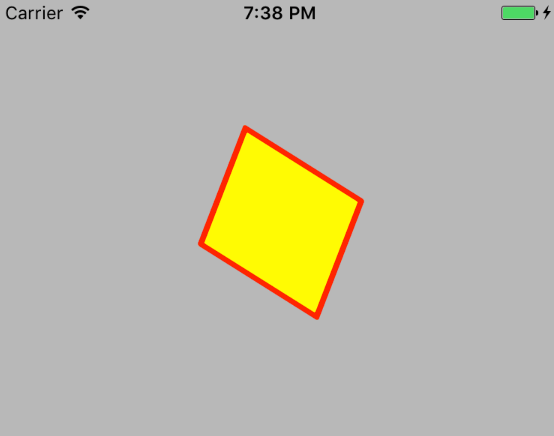# CALayer介绍：2-iOS开发中的坐标系

iOS开发中有两大类型的坐标系，分别为点坐标系(point-based coordinate systems)以及单元坐标系(unit coordinate systems)。另外，当我们对视图进行旋转操作时，还会涉及到iOS中的立体坐标系。

# 点坐标系与单元坐标系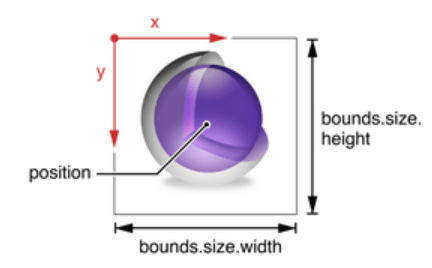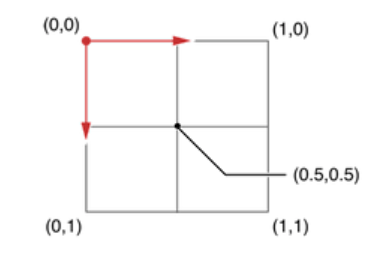# position与anchorPoint

• 位置(positon)。在一个CALayer对象中，位置position是点坐标系中的一个点，该点与锚点anchorPoint是同一个点，或者可以理解为与锚点重合。
``````@property CGPoint position;
``````
• 锚点(anchorPoint)，是单元坐标系中的一个点，默认情况下，它是单元坐标系中的中心点，坐标为(0.5,0.5)。锚点可以形象的理解为一个大头针，把大头针插在一个便签的中心位置，然后再定在墙(点坐标系)上的一个固定位置(position)，这样便签的位置就确定了。
``````@property CGPoint anchorPoint;
``````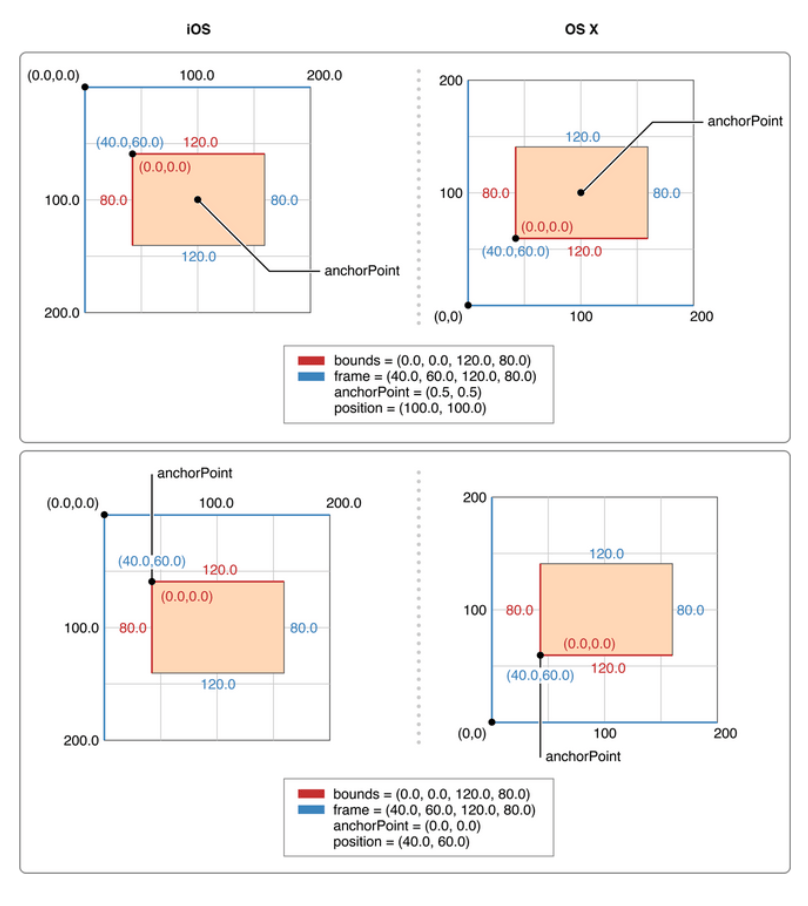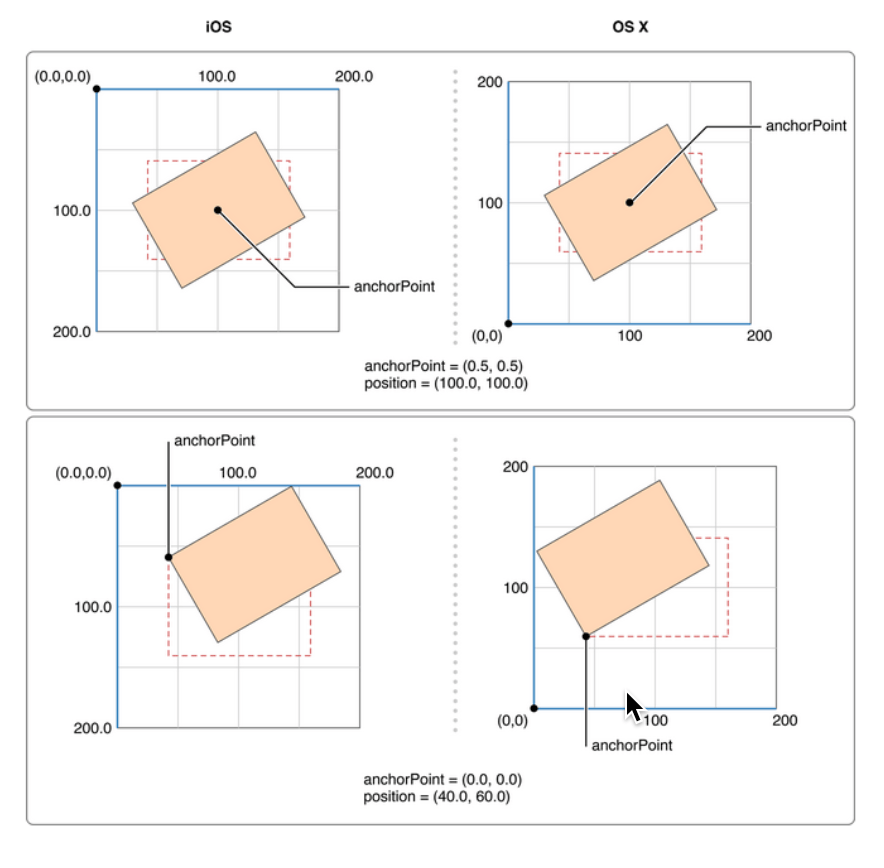# 立体坐标系

iOS开发中还存在三维立体坐标系，除了我们熟知的x轴和y轴之外，还有z轴，z轴以屏幕的左上角为0点，向屏幕外延伸。在CALayer类中，也可以定义与z轴相关的位置属性。

``````@property CGFloat zPosition;
``````
``````@property CGFloat anchorPointZ;
``````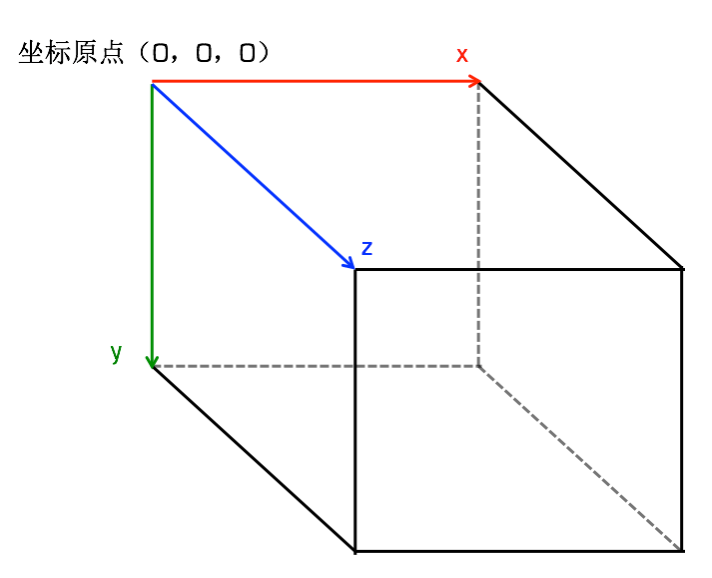``````CA_EXTERN CATransform3D CATransform3DMakeRotation (CGFloat angle, CGFloat x,
CGFloat y, CGFloat z)
``````

• 围绕z轴旋转：
``````subLayer.transform = CATransform3DMakeRotation(M_PI_4, 0, 0, 1);
``````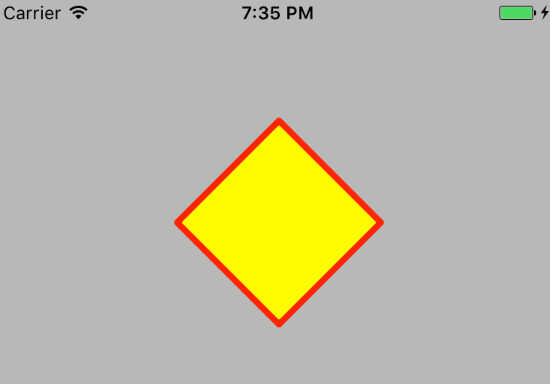• 围绕y轴旋转
``````subLayer.transform = CATransform3DMakeRotation(M_PI_4, 0, 1, 0);
``````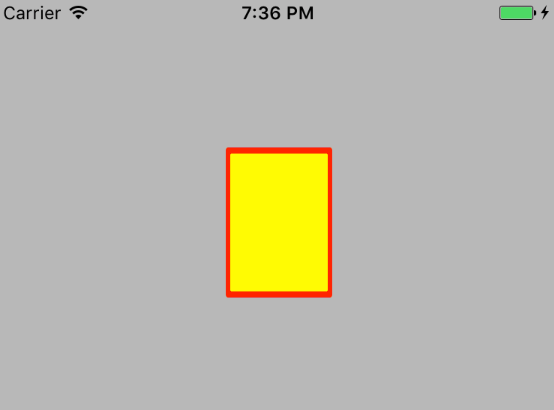• 围绕x轴旋转
``````subLayer.transform = CATransform3DMakeRotation(M_PI_4, 1, 0, 0);
``````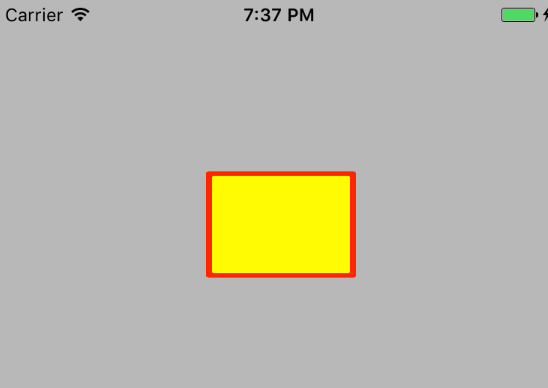• 围绕原点与(1,1,1)连线轴旋转
``````subLayer.transform = CATransform3DMakeRotation(M_PI_4, 1, 1, 1);
``````